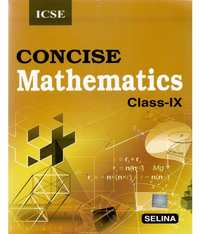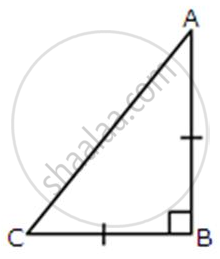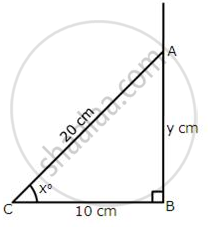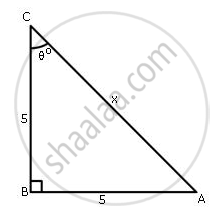# Selina solutions for Concise Mathematics Class 9 ICSE chapter 23 - Trigonometrical Ratios of Standard Angles [Including Evaluation of an Expression Involving Trigonometric Ratios] [Latest edition]

#### Chapters## Chapter 23: Trigonometrical Ratios of Standard Angles [Including Evaluation of an Expression Involving Trigonometric Ratios]

Exercise 23 (A)Exercise 23 (B)Exercise 23 (C)
Exercise 23 (A) [Pages 291 - 292]

### Selina solutions for Concise Mathematics Class 9 ICSE Chapter 23 Trigonometrical Ratios of Standard Angles [Including Evaluation of an Expression Involving Trigonometric Ratios] Exercise 23 (A) [Pages 291 - 292]

Exercise 23 (A) | Q 1.1 | Page 291

find the value of: sin 30° cos 30°

Exercise 23 (A) | Q 1.2 | Page 291

find the value of: tan 30° tan 60°

Exercise 23 (A) | Q 1.3 | Page 291

find the value of: cos2 60° + sin2 30°

Exercise 23 (A) | Q 1.4 | Page 291

find the value of: cosec2 60° - tan2 30°

Exercise 23 (A) | Q 1.5 | Page 291

find the value of: sin2 30° + cos2 30°+ cot2 45°

Exercise 23 (A) | Q 1.6 | Page 291

find the value of: cos2 60° + sec2 30° + tan2 45°

Exercise 23 (A) | Q 2.1 | Page 291

find the value of :
tan2 30° + tan2 45° + tan2 60°

Exercise 23 (A) | Q 2.2 | Page 291

find the value of :

( tan 45°)/ (cos ec30°) +( sec60°)/(co 45°) – (5 sin 90°)/ (2 cos 0°)

Exercise 23 (A) | Q 2.3 | Page 291

find the value of :

3sin2 30° + 2tan2 60° - 5cos2 45°

Exercise 23 (A) | Q 3.1 | Page 291

Prove that:

sin 60° cos 30° + cos 60° . sin 30°  = 1

Exercise 23 (A) | Q 3.2 | Page 291

Prove that:

cos 30° . cos 60° - sin 30° . sin 60°  = 0

Exercise 23 (A) | Q 3.3 | Page 291

Prove that:

cosec2 45°  - cot2 45°  = 1

Exercise 23 (A) | Q 3.4 | Page 291

Prove that:

cos2 30°  - sin2 30° = cos 60°

Exercise 23 (A) | Q 3.5 | Page 291

Prove that:

((tan60°  + 1)/(tan 60°  – 1))^2 = (1+ cos 30°) /(1– cos 30°)

Exercise 23 (A) | Q 3.6 | Page 291

Prove that:

3 cosec2 60°  - 2 cot2 30°  + sec2 45°  = 0

Exercise 23 (A) | Q 4.1 | Page 291

prove that:

sin (2 x 30°) = (2 tan 30°)/(1+tan^2 30°)

Exercise 23 (A) | Q 4.2 | Page 291

prove that:

cos (2 x 30°) = (1 – tan^2 30°)/(1+tan^2 30°)

Exercise 23 (A) | Q 4.3 | Page 291

prove that:

tan (2 x 30°) = (2 tan 30°)/(1– tan^2 30°)

Exercise 23 (A) | Q 5.1 | Page 291

ABC is an isosceles right-angled triangle. Assuming of AB = BC = x, find the value of each of the following trigonometric ratios: sin 45°Exercise 23 (A) | Q 5.2 | Page 291

ABC is an isosceles right-angled triangle. Assuming of AB = BC = x, find the value of each of the following trigonometric ratio: cos 45°Exercise 23 (A) | Q 5.3 | Page 291

ABC is an isosceles right-angled triangle. Assuming of AB = BC = x, find the value of each of the following trigonometric ratios: tan 45°Exercise 23 (A) | Q 6.1 | Page 291

Prove that:
sin 60° = 2 sin 30° cos 30°

Exercise 23 (A) | Q 6.2 | Page 291

Prove that:
4 (sin4 30° + cos4 60°) -3 (cos2 45° - sin2 90°) = 2

Exercise 23 (A) | Q 7.1 | Page 291

If sin x = cos x and x is acute, state the value of x

Exercise 23 (A) | Q 7.2 | Page 291

If sec A = cosec A and 0° ∠A ∠90°, state the value of A

Exercise 23 (A) | Q 7.3 | Page 291

If tan θ = cot θ and 0°∠θ ∠90°, state the value of θ

Exercise 23 (A) | Q 7.4 | Page 291

If sin x = cos y; write the relation between x and y, if both the angles x and y are acute.

Exercise 23 (A) | Q 8.1 | Page 291

If sin x = cos y, then x + y = 45° ; write true of false

• True

• False

Exercise 23 (A) | Q 8.2 | Page 291

secθ . Cot θ= cosecθ ; write true or false

• True

• False

Exercise 23 (A) | Q 8.3 | Page 291

For any angle θ, state the value of : sin2 θ + cos2 θ

Exercise 23 (A) | Q 9.1 | Page 292

State for any acute angle θ whether sin θ increases or decreases as θ increases

• Increases

• Decreases

Exercise 23 (A) | Q 9.2 | Page 292

State for any acute angle θ whether cos θ increases or decreases as θ increases.

• Increases

• Decreases

Exercise 23 (A) | Q 9.3 | Page 292

State for any acute angle θ whether tan θ increases or decreases as θ decreases.

• Increases

• Decreases

Exercise 23 (A) | Q 10.1 | Page 292

If sqrt3 = 1.732, find (correct to two decimal place)  the value of sin 60o

Exercise 23 (A) | Q 10.2 | Page 292

If sqrt3 = 1.732, find (correct to two decimal place)  the value of  (2)/(tan 30°)

Exercise 23 (A) | Q 11.1 | Page 292

Evaluate :
(cos3"A" – 2cos4"A")/(sin3"A" + 2sin4"A") , when A = 15°

Exercise 23 (A) | Q 11.2 | Page 292

Evaluate :

(3 sin 3"B" + 2 cos(2"B" + 5°))/(2 cos 3"B" – sin (2"B" – 10°) ; when "B" = 20°.

Exercise 23 (B) [Page 293]

### Selina solutions for Concise Mathematics Class 9 ICSE Chapter 23 Trigonometrical Ratios of Standard Angles [Including Evaluation of an Expression Involving Trigonometric Ratios] Exercise 23 (B) [Page 293]

Exercise 23 (B) | Q 1.1 | Page 293

Given A = 60° and B = 30°,
prove that : sin (A + B) = sin A cos B + cos A sin B

Exercise 23 (B) | Q 1.2 | Page 293

Given A = 60° and B = 30°,
prove that : cos (A + B) = cos A cos B - sin A sin B

Exercise 23 (B) | Q 1.3 | Page 293

Given A = 60° and B = 30°,
prove that : cos (A - B) = cos A cos B + sin A sin B

Exercise 23 (B) | Q 1.4 | Page 293

Given A = 60° and B = 30°,
prove that : tan (A - B) = (tan"A" – tan"B")/(1 + tan"A".tan"B")

Exercise 23 (B) | Q 2.1 | Page 293

If A =30o, then prove that :
sin 2A = 2sin A cos A =  (2 tan"A")/(1 + tan^2"A")

Exercise 23 (B) | Q 2.2 | Page 293

If A =30o, then prove that :
cos 2A = cos2A - sin2A = (1 – tan^2"A")/(1+ tan^2"A")

Exercise 23 (B) | Q 2.3 | Page 293

If A =30o, then prove that :
2 cos2 A - 1 = 1 - 2 sin2A

Exercise 23 (B) | Q 2.4 | Page 293

If A =30o, then prove that :
sin 3A = 3 sin A - 4 sin3A.

Exercise 23 (B) | Q 3.1 | Page 293

If A = B = 45° ,
show that:
sin (A - B) = sin A cos B - cos A sin B

Exercise 23 (B) | Q 3.2 | Page 293

If A = B = 45° ,
show that:
cos (A + B) = cos A cos B - sin A sin B

Exercise 23 (B) | Q 4.1 | Page 293

If A = 30°;
show that:
sin 3 A = 4 sin A sin (60° - A) sin (60° + A)

Exercise 23 (B) | Q 4.2 | Page 293

If A = 30°;
show that:
(sinA - cosA)2 = 1 - sin2A

Exercise 23 (B) | Q 4.3 | Page 293

If A = 30°;
show that:
cos 2A = cos4 A - sin4 A

Exercise 23 (B) | Q 4.4 | Page 293

If A = 30°;
show that:
(1 – cos 2"A")/(sin 2"A") = tan"A"

Exercise 23 (B) | Q 4.5 | Page 293

If A = 30°;
show that:
(1 + sin 2"A" + cos 2"A")/(sin "A" + cos"A") = 2 cos "A"

Exercise 23 (B) | Q 4.6 | Page 293

If A = 30°;
show that:
4 cos A cos (60° - A). cos (60° + A) = cos 3A

Exercise 23 (B) | Q 4.7 | Page 293

If A = 30°;
show that:
(cos^3"A" – cos 3"A")/(cos "A") + (sin^3"A" + sin3"A")/(sin"A") = 3

Exercise 23 (C) [Pages 297 - 298]

### Selina solutions for Concise Mathematics Class 9 ICSE Chapter 23 Trigonometrical Ratios of Standard Angles [Including Evaluation of an Expression Involving Trigonometric Ratios] Exercise 23 (C) [Pages 297 - 298]

Exercise 23 (C) | Q 1.1 | Page 297

Solve the following equation for A, if 2 sin A = 1

Exercise 23 (C) | Q 1.2 | Page 297

Solve the following equation for A, if 2cos2A = 1

Exercise 23 (C) | Q 1.3 | Page 297

Solve the following equation for A, if sin 3 A = sqrt3 /2

Exercise 23 (C) | Q 1.4 | Page 297

Solve the following equation for A, if sec 2A = 2

Exercise 23 (C) | Q 1.5 | Page 297

Solve the following equations for A, if tan A = 1

Exercise 23 (C) | Q 1.6 | Page 297

Solve the following equation for A, if sqrt3 tan 3 A = 1

Exercise 23 (C) | Q 1.7 | Page 297

Solve the following equation for A, if 2 sin 3 A = 1

Exercise 23 (C) | Q 1.8 | Page 297

Solve the following equation for A, if sqrt3 cot 2 A = 1

Exercise 23 (C) | Q 2.1 | Page 297

Calculate the value of A, if (sin A - 1) (2 cos A - 1) = 0

Exercise 23 (C) | Q 2.2 | Page 297

Calculate the value of A, if (tan A - 1) (cosec 3A - 1) = 0

Exercise 23 (C) | Q 2.3 | Page 297

Calculate the value of A, if (sec 2A - 1) (cosec 3A - 1) = 0

Exercise 23 (C) | Q 2.4 | Page 297

Calculate the value of A, if cos 3A. (2 sin 2A - 1) = 0

Exercise 23 (C) | Q 2.5 | Page 297

Calculate the value of A, if (cosec 2A - 2) (cot 3A - 1) = 0

Exercise 23 (C) | Q 3 | Page 297

If 2 sin x° - 1 = 0 and x is an acute angle;
find :
(i) sin x°
(ii) x°
(iii) cos x and tan x°.

Exercise 23 (C) | Q 4 | Page 298

If 4 cos2 x° - 1 = 0 and 0 ∠ x° ∠ 90°,
find:(i) x°
(ii) sin2 x° + cos2
(iii) (1)/(cos^2xx°) – (tan^2 xx°)

Exercise 23 (C) | Q 5 | Page 298

If 4 sin2 θ - 1= 0 and angle θ is less than 90°, find the value of θ and hence the value of cos2 θ + tan2θ.

Exercise 23 (C) | Q 6.1 | Page 298

If sin 3A = 1 and 0 < A < 90°, find sin A

Exercise 23 (C) | Q 6.2 | Page 298

If sin 3A = 1 and 0 < A < 90°, find cos 2A

Exercise 23 (C) | Q 6.3 | Page 298

If sin 3A = 1 and 0 < A < 90°, find tan^2A - (1)/(cos^2 "A")

Exercise 23 (C) | Q 7 | Page 298

If 2 cos 2A =  sqrt3 and A is acute,
find:
(i) A
(ii) sin 3A
(iii) sin2 (75° - A) + cos2 (45° +A)

Exercise 23 (C) | Q 8.1 | Page 298

If sin x + cos y = 1 and x = 30°, find the value of y

Exercise 23 (C) | Q 8.2 | Page 298

If 3 tan A - 5 cos B = sqrt3 and B = 90°, find the value of A

Exercise 23 (C) | Q 9 | Page 298

From the given figure,
find:
(i) cos x°
(ii) x°
(iii) (1)/(tan^2 xx°) – (1)/(sin^2xx°)
(iv) Use tan xo, to find the value of y.Exercise 23 (C) | Q 10 | Page 298

Use the given figure to find:
(i) tan θ°
(ii)  θ°
(iii) sin2θ° - cos2θ°
(iv) Use sin θ° to find the value of x.Exercise 23 (C) | Q 11.1 | Page 298

Find the magnitude of angle A, if 2 sin A cos A - cos A - 2 sin A + 1 = 0

Exercise 23 (C) | Q 11.2 | Page 298

Find the magnitude of angle A, if tan A - 2 cos A tan A + 2 cos A - 1 = 0

Exercise 23 (C) | Q 11.3 | Page 298

Find the magnitude of angle A, if 2 cos2 A - 3 cos A + 1 = 0

Exercise 23 (C) | Q 11.4 | Page 298

Find the magnitude of angle A, if 2 tan 3A cos 3A - tan 3A + 1 = 2 cos 3A

Exercise 23 (C) | Q 12.01 | Page 298

Solve for x : 2 cos 3x - 1 = 0

Exercise 23 (C) | Q 12.02 | Page 298

Solve for x : cos  (x)/(3)  –1 = 0

Exercise 23 (C) | Q 12.03 | Page 298

Solve for x : sin (x + 10°) = (1)/(2)

Exercise 23 (C) | Q 12.04 | Page 298

Solve for x : cos (2x - 30°) = 0

Exercise 23 (C) | Q 12.05 | Page 298

Solve for x : 2 cos (3x - 15°) = 1

Exercise 23 (C) | Q 12.06 | Page 298

Solve for x : tan2 (x - 5°) = 3

Exercise 23 (C) | Q 12.07 | Page 298

Solve for x : 3 tan2 (2x - 20°) = 1

Exercise 23 (C) | Q 12.08 | Page 298

Solve for x : cos (x/(2)+10°) = (sqrt3)/(2)

Exercise 23 (C) | Q 12.09 | Page 298

Solve for x : sin2 x + sin2 30° = 1

Exercise 23 (C) | Q 12.1 | Page 298

Solve for x : cos2 30° + cos2 x = 1

Exercise 23 (C) | Q 12.11 | Page 298

Solve for x : cos2 30° + sin2 2x = 1

Exercise 23 (C) | Q 12.12 | Page 298

Solve for x : sin2 60° + cos2 (3x- 9°) = 1

Exercise 23 (C) | Q 13 | Page 298

If 4 cos2 x = 3 and x is an acute angle;
find the value of :
(i) x
(ii) cos2 x + cot2 x
(iii) cos 3x (iv) sin 2x

Exercise 23 (C) | Q 14 | Page 298

In ΔABC, ∠B = 90° , AB = y units, BC = (sqrt3) units, AC = 2 units and angle A = x°,
find:
(i) sin x°
(ii) x°
(iii) tan x°
(iv) use cos x°  to find the value of y.

Exercise 23 (C) | Q 15 | Page 298

If 2 cos (A + B) = 2 sin (A - B) = 1;
find the values of A and B.

## Chapter 23: Trigonometrical Ratios of Standard Angles [Including Evaluation of an Expression Involving Trigonometric Ratios]

Exercise 23 (A)Exercise 23 (B)Exercise 23 (C)## Selina solutions for Concise Mathematics Class 9 ICSE chapter 23 - Trigonometrical Ratios of Standard Angles [Including Evaluation of an Expression Involving Trigonometric Ratios]

Selina solutions for Concise Mathematics Class 9 ICSE chapter 23 (Trigonometrical Ratios of Standard Angles [Including Evaluation of an Expression Involving Trigonometric Ratios]) include all questions with solution and detail explanation. This will clear students doubts about any question and improve application skills while preparing for board exams. The detailed, step-by-step solutions will help you understand the concepts better and clear your confusions, if any. Shaalaa.com has the CISCE Concise Mathematics Class 9 ICSE solutions in a manner that help students grasp basic concepts better and faster.

Further, we at Shaalaa.com provide such solutions so that students can prepare for written exams. Selina textbook solutions can be a core help for self-study and acts as a perfect self-help guidance for students.

Concepts covered in Concise Mathematics Class 9 ICSE chapter 23 Trigonometrical Ratios of Standard Angles [Including Evaluation of an Expression Involving Trigonometric Ratios] are Trigonometric Equation Problem and Solution, Trigonometric Ratios of Some Special Angles, Trigonometric Ratios of Some Special Angles, Trigonometric Ratios of Some Special Angles.

Using Selina Class 9 solutions Trigonometrical Ratios of Standard Angles [Including Evaluation of an Expression Involving Trigonometric Ratios] exercise by students are an easy way to prepare for the exams, as they involve solutions arranged chapter-wise also page wise. The questions involved in Selina Solutions are important questions that can be asked in the final exam. Maximum students of CISCE Class 9 prefer Selina Textbook Solutions to score more in exam.

Get the free view of chapter 23 Trigonometrical Ratios of Standard Angles [Including Evaluation of an Expression Involving Trigonometric Ratios] Class 9 extra questions for Concise Mathematics Class 9 ICSE and can use Shaalaa.com to keep it handy for your exam preparation